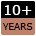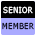Welcome to WebmasterWorld Guest from 18.208.159.25

Forum Moderators: coopster & jatar k

Message Too Old, No Replies

X Y Cordinates

quozt

8:44 pm on Sep 5, 2004 (gmt 0)

Junior Memberjoined:Apr 11, 2004
posts:71

im making a map in which you can select certain places i have given each country certain coridates with x and y e.g. 24,64 6,42 3,33 i am trying to find out how i can auto generate the disance between two points on the map.... and am confused....

any help

Dom

dmorison

9:04 pm on Sept 5, 2004 (gmt 0)

Senior Memberjoined:Apr 3, 2003
posts:1633

If:

Point 1 = x1,y1
Point 2 = x2,y2

Then the distance between Point 1 and Point 2 (in scale units) is the square root of ((x2-x1)2 + (y2-y1)2), which is Pythagoras' Theorm...

[en.wikipedia.org...]

9:14 pm on Sept 5, 2004 (gmt 0)

Junior Memberjoined:Aug 22, 2004
posts:59

Ok heres the math i lernt at school about vector geometry:

first you need the two coordinates of the two points.

Here an example for two points:
A(2�3)
B(4�6)

The vector beween the two points is:
v1 = [[x(B)-x(A)][y(B)-y(A)]] = [[4-2][6-3]] = []

the length of the vector is the square root of the sum of the squares of the the coordinates.

so your distance is:
d = sqrt( (x(v1)^2 + (y(v1))^2 ) = sqrt( (2)^2 + (3)^2 ) = sqrt ( 4 + 9 ) = sqrt( 13 ) = 3,6055512

now all you need to do is put this math in to a php script...
hope i could help

edit: yeah its the same as pythagoras

dmorison

9:21 pm on Sept 5, 2004 (gmt 0)

Senior Memberjoined:Apr 3, 2003
posts:1633

You need the pow() and sqrt() functions to code this in PHP.

With \$x1,\$y1 and \$x2,\$y2 you have:

\$scale_distance = sqrt(pow((\$x2-\$x1),2) + pow((\$y2-\$y1),2));

quozt

10:03 pm on Sept 5, 2004 (gmt 0)

Junior Memberjoined:Apr 11, 2004
posts:71

i would show you my url of the script but outward linking isnt avalible but i use that exact code on my site (i used it before you suggested it) and when i go from a to b it gives me 1 suggestion when i go from b to a it gives me another and when i go from a to a it gives me a completly weird answer because it should be 0!

quozt

10:07 pm on Sept 5, 2004 (gmt 0)

Junior Memberjoined:Apr 11, 2004
posts:71

forget that one of my variables didnt work!

coopster

4:45 am on Sept 6, 2004 (gmt 0)